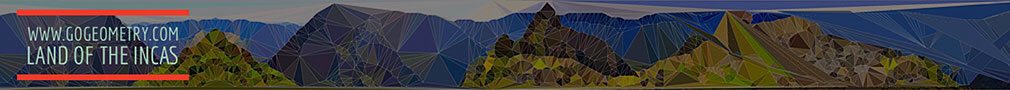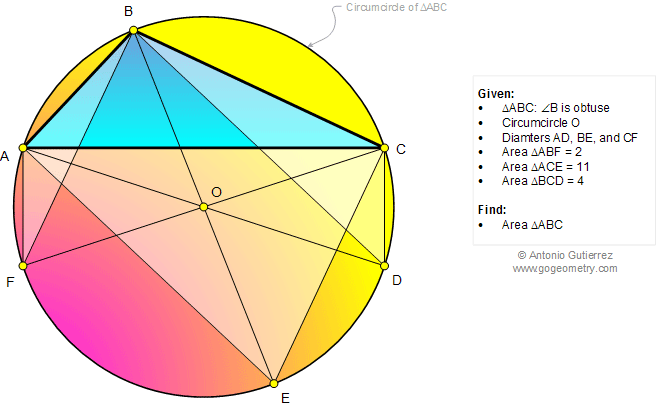# Online Math: Geometry Problem 1033: Triangle, Angle Obtuse, Circumcircle, Diameter, Area. Level: School, College.

< PREVIOUS PROBLEM  |  NEXT PROBLEM >

 The figure below shows a triangle ABC where angle B is obtuse and AD, BE, and CF are diameters of circumcircle O. If the areas of triangles ABF, ACE, and BCD are 2, 11, and 4, respectively, find the area of triangle ABC.Home | SearchGeometry | Problems | All Problems | Open Problems | Visual Index | 10 Problems | Problems Art Gallery Art | 1031-1040 | Triangle | Circle | Circumcenter | Obtuse Angle | Obtuse Triangle | Areas | Triangle area | Diameter | Email | by Antonio Gutierrez

Add a solution to the problem 1033
Last updated: Sep 19, 2014